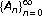Theory and Modern Applications

# Exponential dichotomy of difference equations in l p -phase spaces on the half-line

## Abstract

For a sequence of bounded linear operatorson a Banach space X, we investigate the characterization of exponential dichotomy of the difference equations vn+1 = A n v n . We characterize the exponential dichotomy of difference equations in terms of the existence of solutions to the equations vn+1 = A n v n + f n in l p spaces (1 ≤ p < ∞). Then we apply the results to study the robustness of exponential dichotomy of difference equations.

## References

1. Aulbach B, Minh NV: The concept of spectral dichotomy for linear difference equations. II. Journal of Difference Equations and Applications 1996,2(3):251–262. 10.1080/10236199608808060

2. Bainov DD, Kostadinov SI, Zabreiko PP: Stability of the notion of dichotomy of linear impulsive differential equations in a Banach space. Italian Journal of Pure and Applied Mathematics 1997, 1: 43–50 (1998).

3. Baskakov AG: Semigroups of difference operators in the spectral analysis of linear differential operators. Functional Analysis and Its Applications 1996,30(3):149–157 (1997). 10.1007/BF02509501

4. Chow S-N, Leiva H: Existence and roughness of the exponential dichotomy for skew-product semiflow in Banach spaces. Journal of Differential Equations 1995,120(2):429–477. 10.1006/jdeq.1995.1117

5. Coffman CV, Schäffer JJ: Dichotomies for linear difference equations. Mathematische Annalen 1967,172(2):139–166. 10.1007/BF01350095

6. Dalec'kiĭ JuL, Kreĭn MG: Stability of Solutions of Differential Equations in Banach Space, Translations of Mathematical Monographs. Volume 43. American Mathematical Society, Rhode Island; 1974:vi+386.

7. Henry D: Geometric Theory of Semilinear Parabolic Equations, Lecture Notes in Mathematics. Volume 840. Springer, Berlin; 1981:iv+348.

8. Huy NT: Exponential dichotomy of evolution equations and admissibility of function spaces on a half-line. Journal of Functional Analysis 2006,235(1):330–354. 10.1016/j.jfa.2005.11.002

9. Huy NT, Minh NV: Exponential dichotomy of difference equations and applications to evolution equations on the half-line. Computers & Mathematics with Applications 2001,42(3–5):301–311.

10. Kato T: Perturbation Theory for Linear Operators. Springer, New York; 1980.

11. Latushkin Y, Tomilov Y: Fredholm differential operators with unbounded coefficients. Journal of Differential Equations 2005,208(2):388–429. 10.1016/j.jde.2003.10.018

12. Li T: Die Stabilitatsfrage bei Differenzengleichungen. Acta Mathematica 1934, 63: 99–141. 10.1007/BF02547352

13. Minh NV, Huy NT: Characterizations of dichotomies of evolution equations on the half-line. Journal of Mathematical Analysis and Applications 2001,261(1):28–44. 10.1006/jmaa.2001.7450

14. Minh NV, Räbiger F, Schnaubelt R: Exponential stability, exponential expansiveness, and exponential dichotomy of evolution equations on the half-line. Integral Equations and Operator Theory 1998,32(3):332–353. 10.1007/BF01203774

15. Ngoc PHA, Naito T: New characterizations of exponential dichotomy and exponential stability of linear difference equations. Journal of Difference Equations and Applications 2005,11(10):909–918. 10.1080/00423110500211947

16. Peterson AC, Raffoul YN: Exponential stability of dynamic equations on time scales. Advances in Difference Equations 2005,2005(2):133–144. 10.1155/ADE.2005.133

17. Rodkina A, Schurz H: Global asymptotic stability of solutions of cubic stochastic difference equations. Advances in Difference Equations 2004,2004(3):249–260. 10.1155/S1687183904309015

18. Sell GR, You Y: Dynamics of Evolutionary Equations, Applied Mathematical Sciences. Volume 143. Springer, New York; 2002:xiv+670.

19. Slyusarchuk VE: Exponential dichotomy of solutions of discrete systems. Ukrainskiı Matematicheskiĭ Zhurnal 1983,35(1):109–115, 137.

20. Stefanidou G, Papaschinopoulos G: Trichotomy, stability, and oscillation of a fuzzy difference equation. Advances in Difference Equations 2004,2004(4):337–357. 10.1155/S1687183904311015

## Author information

Authors

### Corresponding author

Correspondence to Nguyen Thieu Huy.

## Rights and permissions

Reprints and Permissions

Huy, N.T., Ngoc Ha, V.T. Exponential dichotomy of difference equations in l p -phase spaces on the half-line. Adv Differ Equ 2006, 058453 (2006). https://doi.org/10.1155/ADE/2006/58453